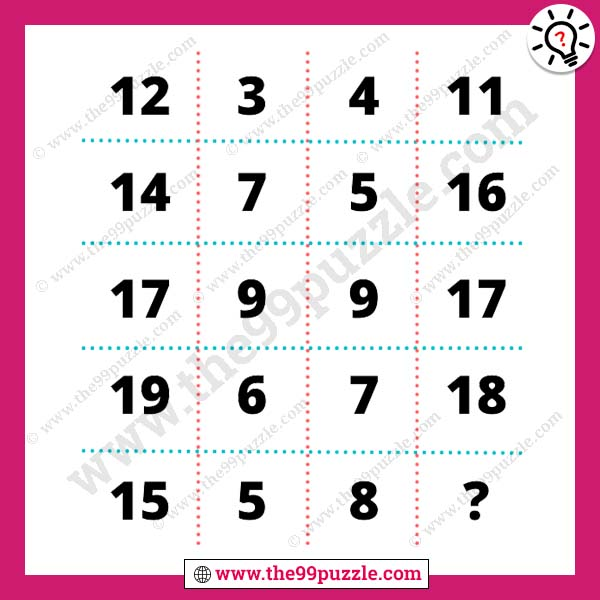# Solve the math and test your logical brain – Puzz251

Can you solve the math and test your logical brain? All high school students accept these types of math puzzle challenges. Math teachers ask these tricky puzzles to their students. This fun and logical math puzzle make you smarter if you practice every day. In this mathematics, you have to find out the math tricks and replace the question mark. Most intelligent students solve math puzzles very quickly if you are a genius at puzzles you can solve this.###### Explanation:

1st Row = 12+(3-4) = 11

2nd Row = 14+(7-5) = 16

3rd Row = 17+(9-9) = 17

4th Row = 19+(6-7) = 18

5th Row = 15+(5-8) = 12